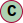# Class ModelBuilder<In, Out>

Model builder to construct and build a Model consisting of graph shapes and services.

## Usage

Models can be created using the ModelBuilder. Once you have added all services and constructed the graph, you can build the model using the `build()` function. A promise will be returned with the created model.

``````import { ModelBuilder } from '@openhps/core';ModelBuilder.create()    .build().then(model => {        // ...    });
``````

The graph shape of a model is immutable and can not be altered after building.

### Shape Builder

Shapes can be created by starting with the `from()` function. This function takes an optional parameter of one or multiple source nodes.

In order to end a shape, the `to()` function needs to be called with one or more optional sink nodes.

``````import { ModelBuilder } from '@openhps/core';ModelBuilder.create()    .from()    .to()    .build().then(model => {        // ...    });
``````

Alternatively for readability with multiple shapes, the shapes can individually be created using the `addShape()` function as shown below.

``````import { ModelBuilder, GraphBuilder } from '@openhps/core';ModelBuilder.create()    .addShape(      GraphBuilder.create()        .from()        .to())    .build().then(model => {        // ...    });
``````

#### Building Source Processors

It is possible to have multiple processing nodes between the source and sink. These processing nodes can manipulate the data frame when it traverses from node to node.

``````import { ModelBuilder } from '@openhps/core';ModelBuilder.create()    .from(...)    .via(new ComputingNode())    .via(new AnotherComputingNode())    .to(...)    .build().then(model => {        // ...    });
``````

#### Helper Functions

Helper functions can replace the `via()` function. Commonly used nodes such as frame filters, merging of data frames from multiple sources, ... can be replaced with simple functions as `filter()` or `merge()` respectively.

``````import { ModelBuilder } from '@openhps/core';import { CSVSourceNode, CSVSinkNode } from '@openhps/csv';ModelBuilder.create()    .from(        new CSVSourceNode('scanner1.csv', ...),        new CSVSourceNode('scanner2.csv', ...),        new CSVSourceNode('scanner3.csv', ...)    )    .filter((frame: DataFrame) => true)    .merge((frame: DataFrame) => frame.source.uid)    .via(new ComputingNode())    .via(new AnotherComputingNode())    .to(new CSVSinkNode('output.csv', ...))    .build().then(model => {        // ...    });
``````

### Debug Logging

When building the model, you can provide a logger callback that has two arguments. An error level complying with normal log levels and a log object that represents an object.

``````import { ModelBuilder } from '@openhps/core';ModelBuilder.create()    // Set the logger that will be used by all nodes and services    .withLogger((level: string, log: any) => {        console.log(log);    })    // ...    .build().then(model => {     // ...    });
``````

Adding services can be done using the `addService()` function in the model builder.

``````import { ModelBuilder } from '@openhps/core';ModelBuilder.create()    .addService(...)    // ...    .build().then(model => {    });
``````

#### Hierarchy-Diagram##### Legendclass

## Properties

graph: ModelGraph<any, any>

## Methods

• Add a service to the model

#### Parameters

• ##### `Optional`proxy: ProxyHandler<any>

Proxy handler

#### Returns ModelBuilder<In, Out>

Model builder instance

• Add multiple services to the model

#### Returns ModelBuilder<In, Out>

Model builder instance

• Add graph shape to graph

#### Parameters

• ##### shape: Model<any, any> | GraphShape<any, any> | GraphBuilder<any, any>

Graph builder or abstract graph

#### Returns ModelBuilder<In, Out>

Current graph builder instance

• Event when graph is ready

#### Parameters

• ##### listener: (() => void | Promise<void>)

Event callback

• (): void | Promise<void>

#### Returns ModelBuilder<In, Out>

• Event before building the graph

#### Parameters

prebuild

• ##### listener: (() => void | Promise<void>)

Event callback

• (): void | Promise<void>

#### Returns ModelBuilder<In, Out>

• Event after building the graph

#### Parameters

postbuild

• ##### listener: ((model) => void | Promise<void>)

Event callback

• (model): void | Promise<void>

• Model logger

#### Parameters

• ##### logger: ((level, message, data?) => void)

Logging function

• (level, message, data?): void

#### Returns ModelBuilder<In, Out>

Model builder instance